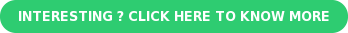Open Cases Aging Report (0-30, 31-60, Over 60 Days)

1. Go to Lists > Search > Saved Searches > New.

2. Select Case.

3. Provide a Search Title.

4. In the Criteria tab > Standard subtab, add filter: Status is none of Closed.

5. In the Results tab > Columns subtab

Select Assign to and make summary type grop.

• Current month select formula numeric and use formula "Decode (Case WHEN (ROUND({today} - {createddate}))= 0 THEN '1' ELSE'0' END, 1,1,0)"
• For 1 – 30 days select formula numeric and use formula “Decode (Case WHEN (ROUND({today} - {createddate})) >= 01 AND (ROUND({today} - {createddate}) <= 30) THEN '1' ELSE '0' END, 1,1,0)”
• For 31-60 days select formula numeric and use formula “Decode (Case WHEN (ROUND({today} - {createddate})) >= 31 AND (ROUND({today} - {createddate}) <= 60) THEN '1' ELSE '0' END, 1,1,0)”
• For 61-90 days select formula numeric and use formula “Decode (Case WHEN (ROUND({today} - {createddate})) >= 61 AND (ROUND({today} - {createddate}) <= 90) THEN '1' ELSE '0' END, 1,1,0)”
• For above 90 days select formula numeric and use formula "Decode (Case WHEN (ROUND({today} - {createddate})) >= 91 THEN '1'ELSE '0' END,1,1,0)"